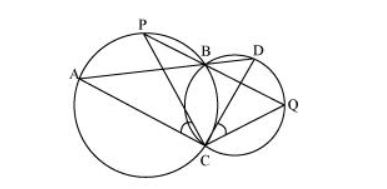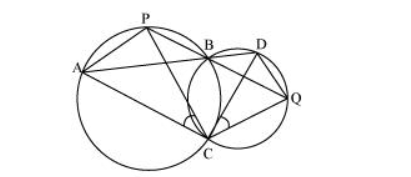# Two circles intersect at two points B and C.

Question. Two circles intersect at two points B and C. Through B, two line segments ABD and PBQ are drawn to intersect the circles at A, D and P, Q respectively (see the given figure). Prove that ∠ACP = ∠QCD.Solution:Join chords AP and DQ.

For chord AP,

$\angle P B A=\angle A C P$ (Angles in the same segment)...(1)

For chord DQ,

$\angle D B Q=\angle Q C D$ (Angles in the same segment) ... (2)

$A B D$ and $P B Q$ are line segments intersecting at $B$.

$\therefore \angle \mathrm{PBA}=\angle \mathrm{DBQ}($ Vertically opposite angles $) \ldots(3)$

From equations $(1),(2)$, and $(3)$, we obtain

$\angle A C P=\angle Q C D$